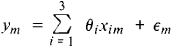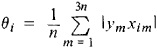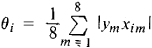# Design of Experiments

(redirected from Completely Randomized Design)
Also found in: Medical.
The following article is from The Great Soviet Encyclopedia (1979). It might be outdated or ideologically biased.

## Design of Experiments

the branch of mathematical statistics dealing with the efficient organization of measurements that are subject to random errors. Experimental design usually conforms to the following scheme. A function f(θ, x) is to be measured with random errors; f(θ, x) depends on unknown parameters (the vector θ) and on variables x that, at the experimenter’s choice, can assume values from some admissible set X. The goal of the experiment is usually (1) to estimate some or all of the parameters or functions of the parameters or (2) to test some hypotheses about the parameters. Given the goal of the experiment, we formulate an optimality criterion for its design. By the design of an experiment we mean a selection of a set of values of the variables x in the experiment. As a rule, the parameters are estimated by the method of least squares and the hypotheses about them are tested by means of Fisher’s F-test, because of the optimal properties of these methods. With respect to the optimality criterion for a design with a given number of experiments, in both cases it turns out to be natural to select a certain function of the variances and the correlation coefficients of the estimates by the method of least squares. When f(θ, x) depends linearly on θ, the optimal design can often be constructed before the experiment is conducted; in other cases, the design is refined during the course of the experiment.

As an illustration, let us consider the determination of three weights θ1, θ2, and θ3 on a two-pan balance if the result of the mth experiment is the difference in weight in the contents of the two pans plus a random error m with mean 0 and variance σ2; that is,where

Xim = (–1)kim

if the ith weight is in the kimth pan in the mth experiment, and xim = 0 if the ith weight is not weighed in the mth experiment. Having weighed each object separately n times (3n experiments), we can estimate the object’s weight by the method of least squares as the quantitywith variance σ2/n. For n = 8, we can achieve the same accuracy after weighing once each the eight different combinations of weights in which each weight lies either in one pan or the other; here, the estimate by the method of least squares is given by the formulawhere i = 1, 2, 3.

The foundations for the design of experiments were laid by the English statistician R. Fisher in a work published in 1935. Fisher emphasized that the efficient design of experiments gives no less important a gain in accuracy than does the optimal processing of the results of measurements. The following branches of the design of experiments can be distinguished.

Factor analysis was historically the first branch. It arose in connection with agrobiological applications of the analysis of variance, a fact reflected in the terminology that has been preserved. Here the function f(θ, x) depends on a vector x of variables, or factors, with a finite number of possible values; the function characterizes the comparative effect of the values of each factor and of combinations of different factors. By algebraic and combinatorial methods there were constructed intuitively attractive designs that simultaneously and in a balanced fashion studied the effect of as great a number of factors as possible. It was subsequently shown that the constructed designs optimized certain natural characteristics of the least squares method of estimation.

The next area of experimental design—the seeking of optimal conditions for the occurrence of various processes—developed under the influence of applications in chemistry and engineering. The methods in this area are essentially modifications of ordinary methods for finding extrema while taking random errors of measurement into account.

There are methods specific to the design of screening experiments. In these experiments it is necessary to isolate the components of the vector x that most strongly affect f(θ, x). This is an important task in the initial stage of an investigation, when the number of components of x is large.

The present-day theory of the design of experiments was developed in the 1960’s. Its methods are closely associated with the theory of approximation of functions and mathematical programming. Optimal designs have been constructed and their properties studied for a broad range of models. Iterative algorithms have been developed that in many cases give satisfactory numerical solutions for problems of experimental design.

### REFERENCES

Hicks, C. R. Osnovnye printsipy planirovaniia eksperimenta. Moscow, 1967. (Translated from English.)
Fedorov, V. V. Teoriia optimal’nogo eksperimenta. Moscow, 1971.

M. B. MALIUTOV

References in periodicals archive ?
This experiment was set up in a completely randomized design with three replicates and fungi growth occurred at a temperature of 28[degrees]C.
In the first experiment, cluster samples (70 g) of both breeding lines were primed in a column bioreactor with 700 ml of -1.0 MPa solutions of potassium nitrate (KNO3), magnesium sulphate (MgSO4), polyethylene glycol - PEG 6000 and polyethylene glycol - PEG 8000 for 6, 12, 24, 48, 72, 96 and 120 hours, hence, experiment consisted of 56 treatments, laid out in completely randomized design. In the second experiment, 70 g samples of clusters of the line AR79 were conditioned with -1.0 MPa solutions of KNO3 and PEG 6000 for 48 hours; hence, priming in the second experiment consisted of two treatments, laid out in completely randomized design.
Data generated for milk production and milk composition for protein, fat, solid not fat (SNF) and total solid (TS) and body weight for seven weeks were analyzed statistically under completely Randomized Design using computer software MINITAB (2000, version 17.0) and the significance of means was compared using the Tukey's test to draw the valid conclusion at certain significance level.
A completely randomized design was followed for this study involving six treatments (0, 50, 100, 150, 200 and 250 kg K2O ha-1) and three repeats.
The treatments consisting of mulching, no mulching and room temperature were arranged in a completely randomized design with three replications.
Therefore, the effect of humidity on fecundity of individual species was determined at each 12 h interval by using analysis of variance (ANOVA) for a completely randomized design and subsequent Tukey's HSD test ([alpha] = 0.05) (SAS Institute 2012).
1997) using statistical analysis package MSTAT-C as a one factor completely randomized design for growth inhibition effect of bakain seeds extract while two factors completely randomized design for toxic effect of the extract.
Three replicated plates were used for each concentration of every fungicide with Completely Randomized Design (CRD).
The trial was executed according to completely Randomized design. The data was analyzed by using variance analysis technique .
The text covers requirements for sound experimentation, completely randomized design, orthogonal contrast for testing hypotheses, and model adequacy checks.
All experimental treatments are being replicated four times in a completely randomized design for a total of 64 plots.
On the basis of a selection index that included total seed yield and 100-seed weight, seed from 14 single OP plants were selected and 58 seedlings from each plant were established in 1992 at the Evans Research Farm, Logan, UT, in a completely randomized design with 58 replications to initiate cycle-2 selection.

Site: Follow: Share:
Open / Close